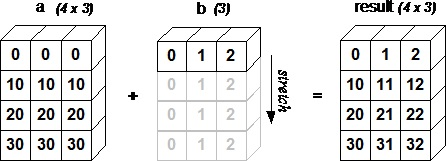# NumPy - 广播

## 示例 1

``````import numpy as np

a = np.array([1,2,3,4])
b = np.array([10,20,30,40])
c = a * b
print c
``````

``````[10   40   90   160]
``````

• `ndim`较小的数组会在前面追加一个长度为 1 的维度。

• 输出数组的每个维度的大小是输入数组该维度大小的最大值。

• 如果输入在每个维度中的大小与输出大小匹配，或其值正好为 1，则在计算中可它。

• 如果输入的某个维度大小为 1，则该维度中的第一个数据元素将用于该维度的所有计算。

• 数组拥有相同形状。

• 数组拥有相同的维数，每个维度拥有相同长度，或者长度为 1。

• 数组拥有极少的维度，可以在其前面追加长度为 1 的维度，使上述条件成立。

## 示例 2

``````import numpy as np
a = np.array([[0.0,0.0,0.0],[10.0,10.0,10.0],[20.0,20.0,20.0],[30.0,30.0,30.0]])
b = np.array([1.0,2.0,3.0])
print  '第一个数组：'
print a
print  '\n'
print  '第二个数组：'
print b
print  '\n'
print  '第一个数组加第二个数组：'
print a + b
``````

``````第一个数组：
[[ 0. 0. 0.]
[ 10. 10. 10.]
[ 20. 20. 20.]
[ 30. 30. 30.]]

[ 1. 2. 3.]

[[ 1. 2. 3.]
[ 11. 12. 13.]
[ 21. 22. 23.]
[ 31. 32. 33.]]
``````